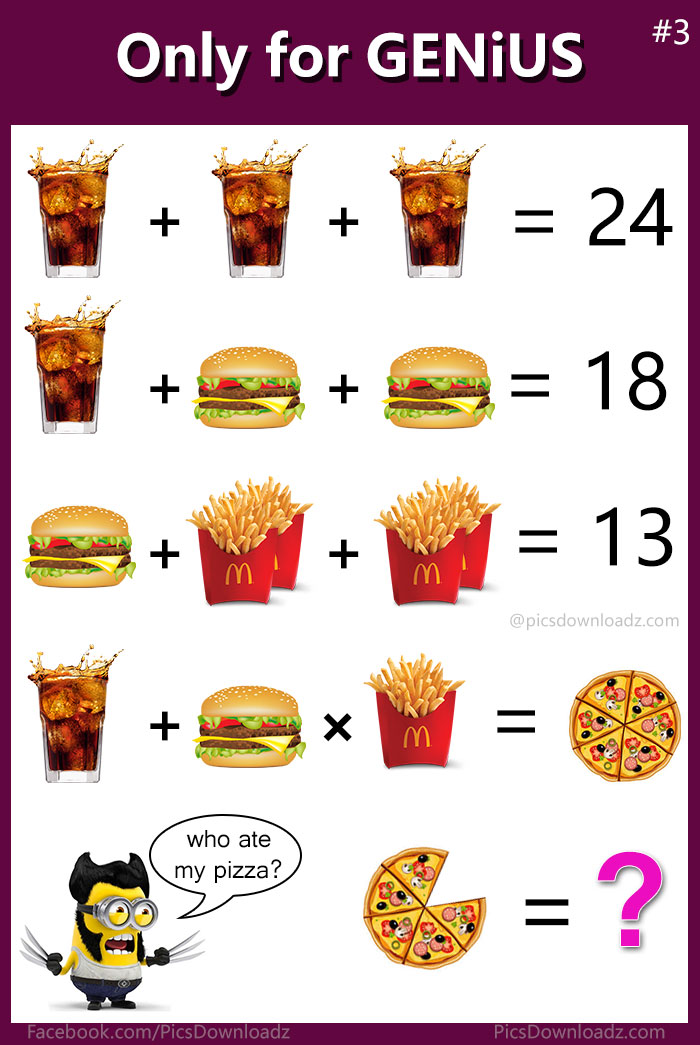This post may contain affiliate links. This means if you click on the link and purchase the item, We will receive an affiliate commission at no extra cost to you. See Our Affiliate Policy for more info.

If Coke + Burger × Fries = Pizza, Then Pizza = ?? Solve this Viral Burger Puzzle

0
18465

Solve this genius burger puzzle. Interesting Burger, Fries and Coke math puzzle. Only for genius math puzzle images with the answer.

Who is hungry? Another tricky foodie brainteaser math puzzle on the way has left people scratching their heads. Solve this confusing burger, coke, and fries puzzle.

Check the combination of the puzzle carefully and find out the value of burger, fries, and coca and then work out the true value of the pizza.

Simple! isn’t it? over to you, best of luck!

Burger, fries and Coke Puzzle Image:Got it? The comment section is all yours. Let see if you crack out the puzzle correctly.

Looking for a hint? Ask the minion 😉 or keep scrolling for the answer.

If your answer is 52, 28, and 26 then you are wrong. And even if it’s 18, you’re close enough but the correct answer is 15.

How? Don’t worry, check out the solution, you will know the trick behind this puzzle.

>> 1st Equation

⇒ Coke + Coke + Coke = 24

⇒ 3Coke = 24

⇒  Coke = 24/3 = 8

⇒ Coke = 8

>> 2nd Equation

⇒ Coke + Burger + Burger = 18  (Put Coke value)

⇒ 8 + Burger + Burger = 18

⇒ Burger + Burger = 18 – 8

⇒ 2 Burger = 10

⇒ Burger = 10/2 = 5

⇒ Burger =  5

>> 3rd Equation

⇒ Burger + Fries + Fries = 13 (Put Burger value from equation 2nd)

⇒ 5 + Fries + Fries = 13

Now here comes the twist, this is the equation which creates a lot of confusion. If you noticed carefully, there are two fries in each section. So going to rewrite the equation;

⇒ 5 + (2Fries) + (2Fries) = 13

⇒ 2 (2Fries) = 13 – 5

⇒ 2 (2Fries) = 8

⇒ (2Fries) = 8/2 = 4

⇒ (2Fries) =  4

⇒ Fries = 4/2 = 2

Therefore, single Fries = 2. Now last equation.

>> Last Equation

Figure out the value of pizza:

⇒ Coke + Burger × Fries = Pizza  (put the values)

⇒ 8 + 5 × 2 = Pizza  (Now do multiplication first)

⇒ 8 + 10 = Pizza

⇒ 18 = Pizza

So we get the value of Pizza = 18 in the fourth equation. But here is another twist, and I’m sure you have noticed! The Pizza is a little bit different “The one slice is missing”. So you have to figure out the value of each slice and find out the true value of the pizza which this puzzle asking for.

The Pizza is already sliced into SIX. So each slice means;

⇒ One Pizza = SIX Sliced = 18

⇒ 6 Sliced = 18

⇒ One Slice = 18/6 = 3

Therefore; Five Sliced Pizza = 5 × 3 = 15

Or Last Pizza = 18 – 3 = 15 (Whatever)

So our final answer is 15.

If you got the right answer, congratulation! your IQ level is high.